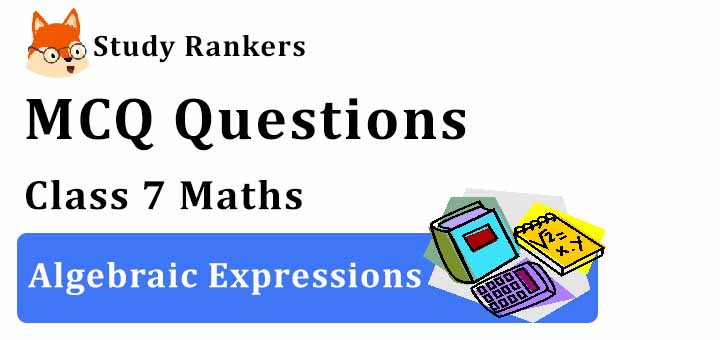## MCQ Questions for Class 7 Maths: Ch 12 Algebraic Expressions1. In a two digit number, the units digit is x and tens digit is (x+3). What is the sum of the digits in the number?
(a) 11x+3
(b) 2x+3
(c) 3+x
(d) 11x+30
► (b) 2x+3

2. The constant term in the expression 1 + x2+ x is
(a) 1
(b) x
(c) x2
(d) None of these
► (a) 1

3. The length and breadth of a rectangular plot are I and b. Two rectangular paths each of width W run inside the plot one parallel to the length and the other parallel to the breadth. What is the total area of the paths?
(a) (l + w)(b + w) − lb
(b) lb − (l − w)(b − w)
(c) (l + b − w)w
(d) lb − (l − 2w)(b − 2w)
► (c) (l + b − w)w

4. Get the algebraic expressions for subtraction of z from y.
(a) y × z
(b) y – z
(c) y + z
(d) y/z
► (b) y – z

5. For what value of 'm' is 9 − 5m = (−1)?
(a) −1
(b) −2
(c) 2
(d) 1
► (c) 2

6. Write an expression : Raju s father s age is 5 years more than 3 times Raju s age. If Raju s age is x years, then father’s age is
(a) 3x – 5
(b) 3x + 7
(c) 5 – 3x
(d) 3x + 5
► (d) 3x + 5

7. When a certain number, 'm' is divided by 5 and added to 8, the result is equal to thrice the number subtracted from 4. What is the value of 'm?
(a) 2
(b) 4/3
(c) −1
(d) 30/7
► (d) 30/7

8. What are the coefficients of y in the expression yz2+ 5?
(a) z
(b) z2
(c) 1
(d) 5
► (b) z2

9. A and B are polynomials and each is the additive inverse of the other. What does it mean?
(a) A = B
(b) A+B is a zero polynomial.
(c) A−B is a zero polynomial.
(d) A−B = B−A
► (b) A+B is a zero polynomial.

10. A _________ can take various values.
(a) variable
(b) expression
(c) term
(d) None of these
► (a) variable

11. What are the coefficients of y in the expression 4x – 3y?
(a) -4
(b) -3
(c) 3
(d) 4
► (b) -3

12. What is the difference between 3a + 2b and −2a − 5b?
(a) 5a+7b
(b) −5a−7b
(c) 5a−7b
(d) a−3b
► (a) 5a+7b

13. The sum of mn + 5 – 2 and mn+3 is
(a) 2mn + 6
(b) mn + 6
(c) 2mn – 6
(d) mn – 6
► (a) 2mn + 6

14. Simplify these expressions and find their values, if x = 3, a = – 1, b = – 2.
3x – 5a – x2 + 9b
(a) -13
(b) 15
(c) 13
(d) None of these
► (a) -13

15. Find the value of x + 4 for x = 2.
(a) 6
(b) 8
(c) 4
(d) None of these
► (a) 6

16. The simplified form of the Boolean expression (X + Y + XY)(X + Z) is
(a) X + Y + Z
(b) XY + YZ
(c) X + YZ
(d) XZ + Y
► (c) X + YZ

17. An expression which contains two unlike terms is called _______.
(a) binomial
(b) monomial
(c) trinomial
(d) None of these
► (a) binomial

18. Simplify combining like terms: 3a – 2b – ab – (a – b + ab) + 3ab + b – a
(a) a - ab
(b) a + ab
(c) a + b
(d) None of these
► (b) a + ab